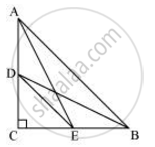# D and E are points on the sides CA and CB respectively of a triangle ABC right angled at C. Prove that AE^2 + BD^2 = AB^2 + DE^2 - Mathematics

D and E are points on the sides CA and CB respectively of a triangle ABC right angled at C. Prove that AE+ BD2 = AB2 + DE2

#### SolutionApplying Pythagoras theorem in ΔACE, we obtain

AC2 + CE2 = AE2 ....(i)

Applying Pythagoras theorem in ΔBCD, we get

BC2 + CD2 = BD2 ....(ii)

Using equations (i) and (ii), we get

AC2 + CE2 + BC2 + CD2 = AE2 + BD2 ...(iii)

Applying Pythagoras theorem in ΔCDE, we get

DE2 = CD2 + CE2

Applying Pythagoras theorem in ΔABC, we get

AB2 = AC2 + CB2

Putting these values in equation (iii), we get

DE2 + AB2 = AE2 + BD2.

Concept: Right-angled Triangles and Pythagoras Property
Is there an error in this question or solution?

#### APPEARS IN

NCERT Class 10 Maths
Chapter 6 Triangles
Exercise 6.5 | Q 13 | Page 151